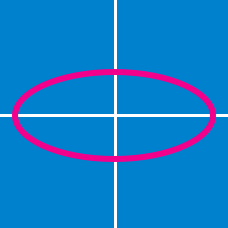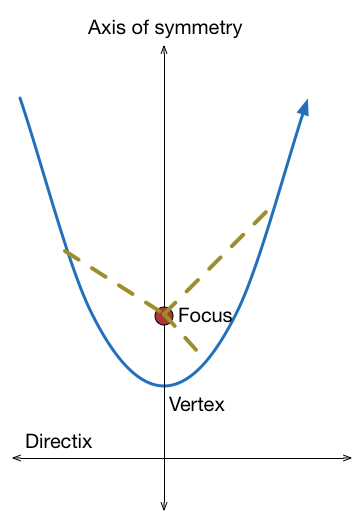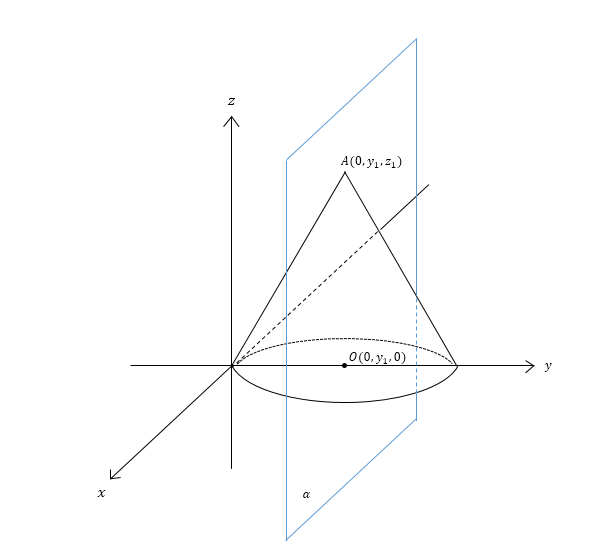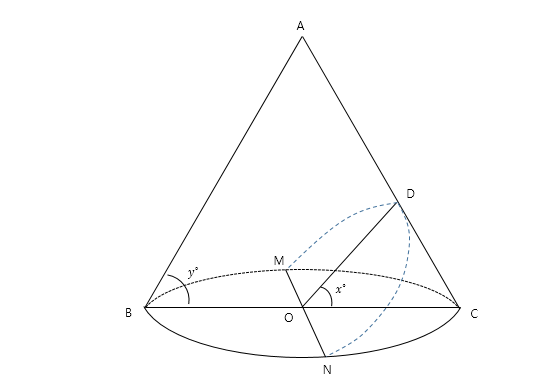Geometry

# Conic Sections - Problem SolvingWhat can we say about light rays that are shot out from the focus of a parabolic mirror, and reflected?In the above diagram, a right cone with vertex $A=(0,16,32)$ and circular base with center $O=(0,16,0)$ is standing on the $xy$-plane such that the origin is an endpoint of a diameter of the circular base. If $\alpha$ is a plane which passes through both $A$ and $O,$ what is the area of the intersection between the cone and the plane $\alpha?$

If $O$ is the origin and $OP$ and $OQ$ are the two tangent lines from the origin to the circle $x^2+y^2-10x+4y+6=0,$ what are the coordinates of the circumcenter of triangle $OPQ ?$The above diagram shows a portion of an infinite right cone and a circular cross section centered at $O$ that is perpendicular to the axis of the cone. Suppose $\angle ABC=60^\circ$ and $\overline{MON}$ is a diameter of the circular cross section. If the shape cut by a plane containing $\overline{MON}$ and a point $D$ on the cone surface is a parabola, what is the value of $x$ in degrees?

For the two sets $A=\left\{ (x,y) \mid x^2+y^2=9 \right\},\ B=\left\{ (0,1), (k,2) \right\}$ on the xy-plane, a set $P=\left\{ (a_1+b_1, a_2+b_2) \mid (a_1, a_2) \in A, (b_1, b_2) \in B \right\}$ represents $2$ circles. If these $2$ circles are externally tangential to each other, what is the value of $k^2$?

×